In mathematics, or more specifically algebra, a quadratic equation is an equation involving only polynomials of the second degree. Quadratic equations are a common part of mathematical solutions to real-world problems in a huge variety of situations. Fortunately, there exists a simple closed formula for finding the roots of such an equation, the quadratic formula, involving no operation more advanced than taking the square root of a real number.

Every polynomial equation can be put into the form:$ax^2+bx+c=0\,$

with a, b and c real and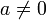$a\not=0$. The quadratic formula specifies the roots of this equation as$x=\frac{-b\pm\sqrt{b^2-4ac}}{2a}\ .$

Here, there are actually two roots being given, although the notation obscures this somewhat. One root is obtained by replacing the "±" appearing in the formula by a "+" sign, and the other is obtained by replacing it with a "−" sign. The formula is guaranteed to work for all quadratic polynomials, but sometimes the roots will be complex numbers even when every other part of the problem deals only with real numbers. Looking at the quadratic formula, we see that the roots will be complex precisely when the discriminant Δ = b2 − 4ac is negative.

There are other less frequently used techniques for solving quadratic equations. Completing the square is just as general as the quadratic formula (in fact, it is used to derive the formula below). Otherwise, if the polynomial has no linear term, or if it is easy to factor, there are faster methods than using the quadratic formula.

In this article, we are assuming as above that the coefficients of the quadratic polynomial are real. Quadratic equations can occur involving many other types coefficients, and the quadratic formula, interpreted correctly, can be applied in many of these other situations as well. See the advanced subpage for information about these more general quadratic equations.

## The problem

Any real second-degree polynomial in the variable x will be of the form$ax^2+bx+c\,$

where a, b, and c are real constants and a is not zero (if it was, the polynomial would only be first-degree). The graph of the function f(x) = ax2 + bx + c is a parabola, and the roots that the quadratic equation will give us are the values of x at which the parabola crosses the x-axis. This means that the roots of the polynomial are the particular values of x for which the polynomial equals zero.

The problem that the quadratic formula solves is to find those roots.

## The solution

The Fundamental Theorem of Algebra tells us that we should expect there to be two roots for a second-degree polynomial, although they might be equal in some cases, and may not be real even when the coefficients are. If we call the roots x + and x then what we are saying is that we have the quadratic equation$ax_\pm^2+bx_\pm+c=0\ .$

This is where the quadratic formula comes in. It tells us that the solutions x + and x can always be found as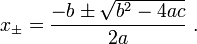$x_\pm=\frac{-b\pm\sqrt{b^2-4ac}}{2a}\ .$

Looking at the above result, it is clear that the discriminant Δ = b2 − 4ac is of interest for two reasons. First it appears in the part of the formula that is either positive or negative depending on whether you are looking at the root x + or x, so it determines whether there are two different roots or just one. Second it is under a square root, so we must wonder what happens when it is negative. This gives us three cases to look at.Figure 2: A parabola with two only one real root, but of multiplicity 2. This corresponds to a second-degree (real) polynomial with a discriminant equal to zero.Figure 3: A parabola with no real roots, as seen by the fact that it does not intersect the horizontal axis. This corresponds to a second-degree (real) polynomial with a negative discriminant.

### Δ > 0

Here we have two distinct real roots, since the square root of Δ will also be real and greater than zero, meaning that we have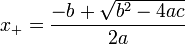$x_+=\frac{-b+\sqrt{b^2-4ac}}{2a}$

and$x_-=\frac{-b-\sqrt{b^2-4ac}}{2a}\ .$

These can be seen graphically as the two red dots in Figure 1. In this case we can rewrite the polynomial in terms of its roots as$ax^2+bx+c = \left(x-x_+\right)\left(x-x_-\right)\ ,$

and it is easy to see that indeed if we set x = x + or x = x then the polynomial will be equal to zero.

### Δ = 0

Now there is only one distinct root. It is still real, and is said to have a multiplicity of 2. This is because the root is given by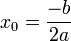$x_0=\frac{-b}{2a}$

and the polynomial can be expressed as$ax^2+bx+c = \left(x-x_0\right)^2\ .$

This case occurs when the parabola described by the polynomial just touches the x-axis at exactly one point, the red dot shown in Figure 2. Again, setting x = x0 in the polynomial makes it vanish.

### Δ < 0

For negative values of the discriminant there are no real roots to the polynomial. Graphically this corresponds to the situation where the lowest point on the parabola is above the x-axis (or the highest point is below it, if a is negative) as shown in Figure 3. In this case the roots still exist, as guaranteed by the fundamental theorem of algebra, but they are complex so cannot be shown on the real number line.

##  Proof

The simplest way to show that the values$x_\pm$ are in fact roots to the polynomial above is to substitute them into the equation\begin{align}ax_\pm^2+bx_\pm+c &=a\left(\frac{-b\pm\sqrt{b^2-4ac}}{2a}\right)^2+b\frac{-b\pm\sqrt{b^2-4ac}}{2a}+c \\ &=\frac{1}{4a}\left(b^2 \mp 2b\sqrt{b^2-4ac}+b^2-4ac\right)-\frac{b^2\mp b\sqrt{b^2-4ac}}{2a}+c \\ &=\frac{b^2\mp b\sqrt{b^2-4ac}}{2a}-c-\frac{b^2\mp b\sqrt{b^2-4ac}}{2a}+c \\ &=0\ , \end{align}

as desired. Notice that this proof is valid even in the case where Δ is less than zero.

##  Derivation of the quadratic formula$ax^2+bx+c=0\,$

and then complete the square. We begin by subtracting c from and then multiplying both sides by 4a, to obtain$4a^2 x^2 + 4abx = -4ac. \,$

As written, the left side does not factor in a useful way. The trick is to add b2 to both sides, because afterward the left side will factor as a complete square:$\left(2ax+b \right)^2= b^2-4ac .\,$

We now take the square root of both sides, remember that there will be two possible square roots for of the number on the right side, indicated by the$\pm$ symbol: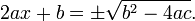$2ax + b = \pm \sqrt{b^2-4ac}.$

Finally, we solve for x to obtain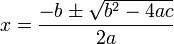$x = \frac{-b \pm \sqrt{b^2-4ac}}{2a}$,Some content on this page may previously have appeared on Citizendium.